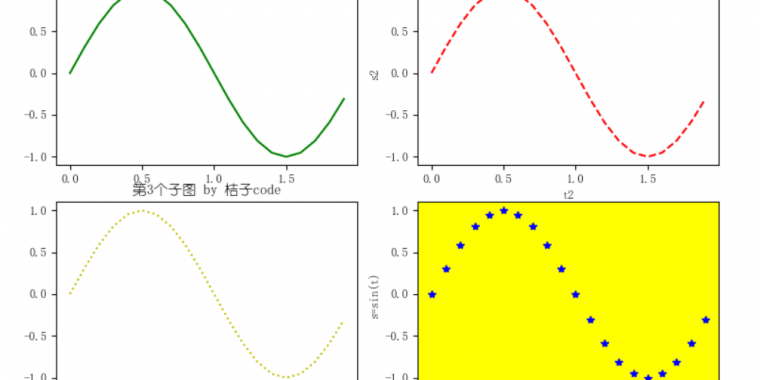# 数据可视化~matplotlib默认配置修改（可解决中文、负号等显示问题）``````import numpy as np
import matplotlib.pyplot as plt

if __name__ == '__main__':
x = np.arange(-3,5)
y = x*x*x
plt.title("桔子code")
plt.xlabel("x")
plt.ylabel("y")
plt.plot(x,y)
plt.show()``````

``````>>> import matplotlib
>>> matplotlib.matplotlib_fname()
'D:\\Python\\Python38\\lib\\site-packages\\matplotlib\\mpl-data\\matplotlibrc'``````

``````font.family:  YouYuan
#font.style:   normal
#font.variant: normal``````

``````if __name__ == '__main__':
plt.rcParams['font.family'] =  ['YouYuan']
x = np.arange(-3,5)
y = x*x*x
plt.title("桔子code")
plt.xlabel("x")
plt.ylabel("y")
plt.plot(x,y)
plt.show()``````

``````    plt.rcParams['font.family'] =  ['YouYuan']
plt.rcParams['axes.unicode_minus'] = False  ``````

``plt.rc('font',family='YouYuan',size='15')``

plt.rc()方法的第1个参数为‘axes’，入参unicode_minus =’False’就能正常显示负号。

``    plt.rc('axes',unicode_minus='False')``

``````print('\n-----欢迎来到juzicode.com')
print('-----公众号: 桔子code/juzicode \n')
import numpy as np
import matplotlib.pyplot as plt

if __name__ == '__main__':
plt.rc('font',family='Youyuan',size='15')
plt.rc('axes',unicode_minus='False')
x = np.arange(-3,5)
y = x*x*x
plt.title("桔子code")
plt.xlabel("x")
plt.ylabel("y")
plt.plot(x,y)
plt.show()``````

``````import numpy as np
import matplotlib.pyplot as plt

if __name__ == '__main__':
plt.rc('font',family='Youyuan',size='15')
plt.rc('axes',unicode_minus='False')
plt.rc('axes',linewidth='5.0',grid='True')
x = np.arange(-3,5)
y = x*x*x
plt.title("桔子code")
plt.xlabel("x")
plt.ylabel("y")
plt.plot(x,y)
plt.show()``````

``````#xtick.major.size:    3.5     # major tick size in points
#xtick.minor.size:    2       # minor tick size in points``````

``    plt.rc('xtick.major',width='5',size='12')``

```附录~常用字体：
华文楷体：STKaiti
华文宋体：STSong
华文细黑：STHeiti Light [STXihei]
华文黑体：STHeiti
华文仿宋：STFangsong
苹果俪中黑：Apple LiGothic Medium
苹果俪细宋：Apple LiSung Light
俪黑 Pro：LiHei Pro Medium
俪宋 Pro：LiSong Pro Light
标楷体：BiauKai

微软正黑体：Microsoft JhengHei
微软雅黑体：Microsoft YaHei
仿宋_GB2312：FangSong_GB2312
楷体_GB2312：KaiTi_GB2312
标楷体：DFKai-SB
黑体：SimHei
宋体：SimSun
新宋体：NSimSun 新细明体：PMingLiU
细明体：MingLiU
仿宋：FangSong
楷体：KaiTi
隶书：LiSu
幼圆：YouYuan
华文彩云：STCaiyun
华文琥珀：STHupo
华文隶书：STLiti
华文行楷：STXingkai
华文新魏：STXinwei
华文细黑：STXihei
华文楷体：STKaiti
华文宋体：STSong
华文中宋：STZhongsong
华文仿宋：STFangsong
方正舒体：FZShuTi
方正姚体：FZYaoti
```